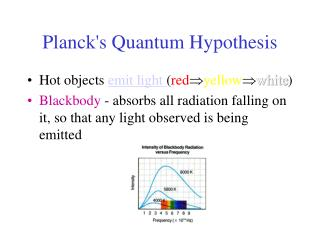# Planck's Quantum Hypothesis - PowerPoint PPT PresentationDownload PresentationPlanck's Quantum Hypothesis

Planck's Quantum HypothesisDownload Presentation## Planck's Quantum Hypothesis

- - - - - - - - - - - - - - - - - - - - - - - - - - - E N D - - - - - - - - - - - - - - - - - - - - - - - - - - -
##### Presentation Transcript

1. Planck's Quantum Hypothesis • Hot objects emit light (redyellowwhite) • Blackbody - absorbs all radiation falling on it, so that any light observed is being emitted

2. Electromagnetic wave theory predicted that objects would emit radiation, but did not accurately predict the observed spectrum of light at higher frequencies (ultraviolet catastrophe) • In 1900, Max Planck suggested that the energy of vibration of the atoms in a solid is not continuous but can only have discrete values given by E=nhf • where h is Planck's Constant and has been found experimentally to be 6.626 X 10-34 Js

3. Using these discrete energy values of hf, Planck was able to fit a mathematical equation to the entireblackbody curve. • Revolutionary Idea: Energy exists only in discrete amounts!!!!! • Smallest amount of energy possible (hf) is called a quantum of energy. • Planck himself was not entirely happy with this idea, but this was in fact the birth of modern physics.

4. Photoelectric Effect • Photoelectric Effect- when light shines on a metal surface, electrons can be emitted from the surface. Only light above a certain frequency will cause this affect to happen; • Wave theory does not accurately explain photoelectric effect. • Einstein extended Planck's quantum theory to light in 1905 --- since all light ultimately comes from a radiating source, light may be transmitted as tiny packets (photons), each with an energy of hf

5. Einstein's Photoelectric Theory • one electron can be ejected upon collision with one photon, with the photon losing all of its energy • some minimum energy Wo (called the work function) is required to release the electron • if hf > Wo, the electron will be released. The maximum energy of the electron will be given by KEmax = hf – Wo (for electrons buried deeper in the metal, more energy than Wo may be needed)

6. Predictions of Einstein's Theory • an increase in intensity means more photons hitting the metal, which should mean more electrons being released; the kinetic energy of each electron should not be changed • if f < fo(where fo is the threshold frequency and hfo=Wo), no electrons will be released • if f is increased, KEmax increases linearly

7. Einstein's predictions were verified by Millikan by 1916. • Energy is often expressed in electron volts (eV). The electron volt is a smaller unit of energy than a joule 1 eV = 1.6 X 10-19 J • The Photoelectric Effect provided the first evidence for a particle theory of light.